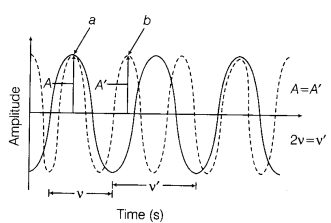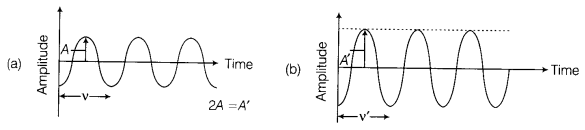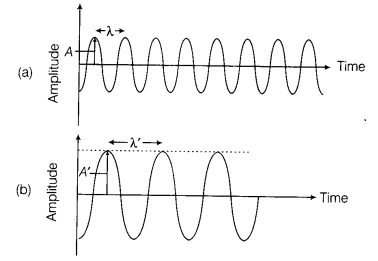# One diagram showing two sound waves having the same amplitude but different frequencies

Represent graphically

1. one diagram showing two sound waves having the same amplitude but different frequencies.
2. two separate diagrams showing sound waves having the same frequency but different amplitudes.
3. two separate diagrams showing sound waves having different amplitudes and also different wavelengths.
1. A diagram showing two sound waves having the same amplitude (A and A’) but different frequencies (v and v’).2. Two separate diagrams showing sound waves having the same frequency (v and v’) but different amplitudes (A and A’), i.e. A ≠ A’3. Two separate diagrams showing sound waves having different amplitudes (A and A’) and also different wavelengths (λ and λ’), i.e. A ≠ A’ and λ ≠ λ’.